This article uses embedded SP fonts (SPIonic and SPDoric) for non-Roman text. Another version which uses Unicode is located here.

## J. C. Thorpe

### Abstract

The use of multivariate statistics for the analysis and classification of New Testament manuscripts is examined, the problem of coding the manuscripts for statistical analysis is considered, and various coding schemes are presented. The operation and suitability of a number of statistical techniques for manuscript classification is also discussed.

### Introduction

1. This article considers the statistical techniques available for use in the classification of New Testament manuscripts. The techniques described and evaluated are not novel, but are used routinely by statisticians for a number of purposes. They are implemented on computers in a wide variety of general purpose statistical packages.

2. The process of classifying New Testament manuscripts aims to identify groups and subgroups of manuscripts with similar characteristics. Statistical analysis will not guarantee a full genealogical classification of the available manuscripts; at best it will show the degree to which manuscripts are related and will highlight apparent clusters of manuscripts.

3. New Testament manuscripts contain many variation units. In this paper, a variation unit is referred to as a locus. Each locus has at least two possible readings; indeed, in some cases there can be six or more different readings for the same locus. Every manuscript has one reading at each locus. One of the major problems of analysis is that for many manuscripts there are lacunae. The full range of readings at many of the loci is therefore not known.

### Why Use Statistical Techniques?

4. Manuscripts are the product of transcription by copyists, whose errors were the result of human failings. It may thus seem odd to use mathematical techniques to analyse the copyists' work; however, the sheer number of variations makes it effectively impossible for the unaided human mind to carry a full representation of the variations for even a single book. For this reason, a formal method of tracking and investigating the variation has obvious merits.

5. Statistical methods for investigating manuscript relationships have the following advantages:

1. 6. Objectivity: A statistical technique will produce the same result no matter who carries it out. It cannot be affected by the preconceptions of the person who applies the technique, although a scholar could apply an inappropriate technique or interpret the outcome of the technique incorrectly. The results of statistical analysis are repeatable.

2. 7. Rigour: The results of statistical analysis are achieved through an open set of logical steps which can be inspected by anyone who wishes to do so. Assumptions are stated as part of the analysis and the effect of changing these assumptions can be investigated.

3. 8. Comprehensiveness: Statistical techniques can, by using computers, handle very large quantities of data; a large study can be carried out almost as easily as a small one.

9. Scholars using non-statistical methods strive to produce results with these characteristics. When appropriate statistical techniques are used, these features are an automatic result of the analysis.

10. There are, however, limits to the use of statistical methods. They can only test hypotheses that have already been formulated by human beings. Human imagination is required to devise questions that can be expressed in rigorous terms and investigated statistically. There can also be a problem with the interpretation of the results of statistical analysis; the output of a statistical procedure may need human interpretation to decide its significance in terms of textual criticism.

### Types of Data

11. Whether or not a statistical technique is appropriate depends on the kind of data to be analysed. This section introduces the kinds of data encountered in statistical analysis.

1. 12. Categorical data (also known as nominal data): In data of this kind, the value assigned to a variable has no meaning other than to indicate a particular fact associated with the object. In particular, categorical data cannot be used to provide a natural order for observations. For example, consider the manuscripts A, B, and C. One variable which could be associated with these manuscripts is current location (A is in London, B is in the Vatican, and C is in Paris). One could code this data as 1 = London, 2 = Vatican, and 3 = Paris, but the values 1, 2, and 3 are purely arbitrary. One cannot use the codes to order the data in any meaningful way; that is, one cannot conclude that Paris is "more than" London because its code has a higher value.

2. 13. Ordinal data: Data of this kind can be used to place observations in order, but the magnitude of the data has no absolute meaning. For example, consider manuscripts A, B, and E. B is the oldest, A is the second oldest, and E is the youngest. One could code these facts by assigning the value 1 to the oldest manuscript, 2 to the middle manuscript, and 3 to the most recent. Sorting the manuscripts into numerical order based on this variable would place them in age order, but no further information can be gained from the code. There is a similarity between ordinal data and nominal data in that neither can be used for arithmetic calculation. (Some class both nominal and ordinal data as categorical data, but most statisticians use the term categorical data to refer to nominal data alone.)

3. 14. Cardinal data: For data of this kind, the magnitude is meaningful and one can derive useful information by subtracting two values. For example, manuscript dates are cardinal data and subtracting two dates produces a meaningful result.

4. 15. Absolute data: Some cardinal measures are absolute. When a measure is absolute, the ratio between two values is meaningful as well as the difference. For example, consider manuscripts B (4th century) and 075 (10th century). The date is not absolute; dividing the date of one manuscript by the date of the other has no meaning, but one can calculate that B is six centuries older than 075 by subtraction. Thus the date is a cardinal measure. The age of B is about 16 centuries and that of 075 about 10 centuries. This is an absolute measure, and one can calculate that B is about 1.6 times older than 075. That is, the age of a manuscript is an absolute measure.

#### Binary Measures

16. Categorical data with more than two possible states is sometimes called multistate data. By contrast, a binary measure can adopt only two values, typically zero or one. Any discrete measure can be represented as a combination of binary measures without loss of information. Nevertheless, care needs to be taken when analysing data that has been recoded as binary variables.

17. The most common use of binary variables is as an alternative representation of categorical data. When this is done, a categorical variable V with n states (v1, v2, ... , vn) is coded as n binary variables B1, B2, ... , Bn such that:
Bk = 1 if V = vk and
Bk = 0 if V ≠ vk.

18. In this representation, only one of the binary variables B1, B2, ... , Bn may have the value one at any given time. This requirement is represented by the constraint: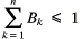19. One of the problems with using a binary representation of data is that each variable transformed into a set of binary variables introduces another constraint into the formal system representing the data.

#### Example

20. Using the code 1 = London, 2 = Vatican, and 3 = Paris, the current locations of manuscripts A, B, and C can be denoted by the variable LOCk, where k ∈ {A, B, C}. The data can be recoded as the set of binary variables LONk, VATk, and PARk with the added constraint that LONk + VATk + PARk = 1:

 k LOCk LONk VATk PARk A 1 1 0 0 B 2 0 1 0 C 3 0 0 1

#### Objects and Variables

21. Multivariate statistics makes use of the concepts of object and variable. Multivariate data consists of observations made on a set of objects using a number of variables--hence the term 'multivariate'. An object is an entity which is to be described or classified in the analysis; in the statistical analysis of manuscripts, the objects being analysed are usually the manuscripts themselves. A variable is a measure that varies from object to object. One might use the readings at a particular set of loci as variables to describe a set of manuscripts.

### Coding Manuscripts for Statistical Analysis

22. A collation lists manuscript texts in a parallel fashion to facilitate comparison of their readings. The usual procedure is to list readings that vary from a base text such as the Textus Receptus or the United Bible Societies Greek New Testament. A less common alternative is to print the texts in parallel columns.

23. For the purposes of statistical analysis, it is necessary to recode manuscript collation data into a form that can be handled by a statistical package. Usually, this means coding the readings as numerical values. The simplest method assigns a single categorical variable to each locus in the text at which variant readings occur. Each reading for the locus is then assigned an arbitrary and unique numerical value. The reading of any given manuscript at this locus is then represented by the corresponding numerical value.

#### Example

24. The fourth word of John 1:4 in UBS4 is h(=n. There are two other variants: some witnesses (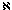D and others) have e)stin, while Wsupp omits the word altogether. One could code the variants as: 1 for h(=n, 2 for e)stin, and 3 for the omission. Using this coding scheme, the respective values of the data variables for manuscripts D, Wsupp, and B at this locus are 2, 3, and 1. Using mathematical notation, one might define a data matrix Dataj,k , where index j denotes the locus and index k denotes the manuscript. If this locus is assigned the index 2, we would write that Data2,D = 2, Data2,Wsupp = 3, and Data2,B = 1.

25. It can be difficult to decide what constitutes a locus and what constitutes a variant reading. Taking the start of the Lord's prayer at Luke 11:2 as an example, one might feel that the words form a single sense unit and that this should be counted as a single locus. However, another might decide that the words form several logical units and that there should therefore be several different loci, as in the UBS4 apparatus. Whether variants are coded as one locus or several is one of the subjective elements of statistical analysis.

26. A locus where more than one variant exists could, of course, be coded as a family of binary variables with an added constraint. It is important at this stage to include a further binary variable to indicate whether the manuscript is extant at this particular locus or whether there is a lacuna; where one of the readings is a known omission from the text, a binary variable should be set up to register the fact. The binary representation should be the same whether it is coded directly from the text or is generated from an earlier categorical representation.

27. A coded description of a manuscript can be thought of as a multidimensional vector of values indicating a single point in a vector space (manuscript space), with each manuscript having a corresponding point in the space. However, there are problems with this picture when each locus is described by a single categorical variable and where some of the loci have more than two readings. In such a situation, the value of the variable at the locus does not represent the magnitude of a displacement in manuscript space, which is the underlying assumption of the vector representation. Unfortunately, many multivariate techniques assume this model of data representation.

28. This problem can be alleviated to some extent by coding loci with more than two variants as sets of binary variables. However, even this is far from an ideal solution as each locus thus represented implies a constraint. Coding an appreciable portion of text can be expected to add many tens of such constraints (or even hundreds), meaning that some areas of manuscript space are infeasible; they cannot be occupied by a manuscript, even in theory. As most multivariate techniques assume that data is continuous and cardinal with no forbidden regions, the use of these techniques must be considered suspect for both binary and multistate representations.

### Similarity and Dissimilarity matrices

29. One convenient and theoretically acceptable method of expressing the degree of similarity between pairs of manuscripts within a set of manuscripts being compared is the similarity matrix S. An element si,j of this matrix contains the number of loci where manuscript Zi has the same reading as manuscript Zj, divided by the number of loci where both texts are extant.

30. The similarity matrix S is square, with one row and one column for each manuscript under investigation. The diagonal elements si,i all have the value one because they represent the similarity of a manuscript to itself. Also, S is symmetric: si,j = sj,i .

31. Some multivariate statistical techniques can operate from a dissimilarity matrix D. This is closely related to the similarity matrix S: every element di,j of D has the value 1 - si,j, where si,j is the corresponding element of S.

32. It is relatively straightforward to construct a similarity matrix from coded data in which each locus corresponds to a single variable. Construction involves working through all the loci under investigation, counting the number of loci ni,j for which both manuscripts Zi and Zj are extant, and the number of loci mi,j for which both manuscripts have the same reading. The similarity value si,j is given by the ratio of the two values:33. There can be problems in calculating a correct value for ni,j when the manuscripts have been coded using binary variables. The only way to be certain of reaching a correct value is to include an additional binary variable for each locus and each manuscript, indicating whether the text exists there. If this variable is used, mi,j is the number of variables where both manuscripts contain the value one and ni,j is the number of loci at which both manuscripts are marked as extant.

34. Note that the number of occasions when two manuscripts both register a zero must not be counted in a binary representation as this will increase the weighting given to loci with more variants. Otherwise, the analysis will consider a locus with, say, four variants to be twice as important as a locus with two, and manuscripts may appear similar for no reason other than that they have lacunae in the same places.

35. It is also important not to mix representations. If a binary representation is used for some loci it, must be used for all loci. Mixing representations confounds the counting procedures used to generate a similarity matrix and produces incorrect results.

### Equivalence of Dissimilarity Matrices

36. Codex Sinaiticus is thought to change character from "Western" to "Alexandrian" part way through the Gospel of John1. Is this apparent change of textual character real or can it be reasonably attributed to a random fluctuation in affiliations? Another interesting question is whether a supplement such as Wsupp has the same character as the original MS. Such questions can be investigated by performing statistical tests on dissimilarity matrices generated from manuscript texts.

37. The Mantel test is used to investigate whether two dissimilarity matrices are the same. It tests the hypotheses:

H0: there is no relationship, and
H1: there is a relationship between the two matrices.

38. Typical manuscript data do not fit well with the underlying model of this test, where the null hypothesis (i.e. H0) assumes no relation whatsoever between the respective dissimilarity matrices. The question of relationship may be better answered by applying repeated tests on the value of a proportion using univariate statistics.

### Principal Component Analysis

39. The purpose of principal component analysis (PCA) is to describe a set of multivariate data in terms of a set of uncorrelated variables, each of which is a linear combination of the original variables. The new variables are called Principal Components. These are listed in decreasing order of importance so that the first principal component accounts for as much as possible of the variation of the original data, the second principal component accounts for as much as possible of the remaining variation, and so on. The process continues until the number of principal components generated equals the number of original variables in the data. The hope is that the first few principal components will account for a large proportion of the variation so that the objects can be well described using a small number of variables.

40. Some regard PCA as an effective means to investigate manuscript relationships. For example, Wieland Willker2 performed PCA on the first chapters John's Gospel. He indicates that plotting the first two principal components reveals textual clusters which correspond to known text types.

41. Nevertheless, there are problems associated with using PCA to classify manuscripts:

• it assumes a space comprised of cardinal variables whereas manuscript variants are represented by categorical variables. The mismatch can be overcome to some extent by using a binary coding scheme; however, this still leaves problems with forbidden regions, loss of detail, and the introduction of random error.
• it has no simple means of coping with missing values. As many manuscripts contain lacunae, PCA is not likely to produce a reasonable description of more than a small proportion of manuscripts without a strategy to handle missing values.

42. Willker avoids these pitfalls by only using loci for which none of the manuscripts he is investigating have lacunae. His work suggests that there is some value in using PCA to investigate manuscript relationships. However, general use of the technique is limited to places where no lacunae are present in the set of MSS under investigation. In any event, there remain the problems of non-cardinal data and implied constraints.

### Factor Analysis

43. Like PCA, factor analysis attempts to describe the full set of p variables X1, X2, ... , Xp in terms of a linear combination of other variables. Unlike PCA--which retains the original number of variables in its results--factor analysis is restricted to a smaller number q of variables F1, F2, ... , Fq, this number being specified by the analyst at the beginning. The new variables are known as factors.

44. The model employed by factor analysis is: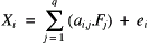The coefficients ai,j are called the factor loadings and ei is called the error term. It represents a factor which is specific to the variable Xi. The data is usually normalized so that each Xi has a mean of zero and variance of one. Once normalization has been carried out, the error terms also have a mean of zero.

45. Factor analysis attempts to choose factors and factor loadings so that the factors are mutually unrelated and the variation described by the error terms is minimised. Very often the process will start with factors which are the first q principal components and then gradually alter these to minimise the contribution of the error terms; this process is called "rotating the factors". The final factors are frequently, but not always, close to the first few principal components.

46. Factor analysis requires all the assumptions of PCA and therefore inherits all of its problems when applied to manuscript data. Factor analysis does its best to represent the data with the allocated number of dimensions. Because of this it may provide a better fit than PCA where the map produced must have a restricted number of variables.

### Multidimensional Scaling

47. Multidimensional scaling treats the dissimilarity matrix as a distance matrix which describes the geography of a space containing the objects under investigation. It generates a set of object coordinates that conform to these 'distances' as closely as possible, thereby allowing the objects to be plotted on a map.

48. There are two varieties of multidimensional scaling. The first, called classical scaling, generates the coordinates using an algebraic procedure that assumes that the 'distances' are Euclidean, although it is robust against departures from this assumption3. The coordinates have the same number of dimensions as the original data. As with PCA, the first few dimensions are the most significant. Indeed, the results of PCA and classical scaling are equivalent under certain circumstances4. Classical scaling has already been used to investigate relationships among New Testament manuscripts5.

49. The second variety, called non-metric multidimensional scaling or ordinal scaling, seeks to find object coordinates that conform to the rank order of the 'distances' rather than their Euclidean values. This technique is therefore preferable in situations where the Euclidean assumption is suspect. The number of dimensions for the coordinates can be specified in advance. Two- or three-dimensional representations are popular because the resulting coordinates can be readily plotted on conventional maps.

50. The process attempts to minimize the following stress measure:where n is the number of objects in the investigation
di,j is the distance between objects i and j recorded in the dissimilarity matrix, and
i,j is the distance between objects i and j calculated from the generated coordinates.

51. The resulting map is considered to be a good representation of the data if the stress value is less than 5%, but a solution for which the value is less than 10% might be considered acceptable in some circumstances. It is, however, unwise to depend entirely on this measure6. A plot of the stress versus the number of dimensions in the map can help to show the number of variables required to provide an adequate description of the data.

52. A successful multidimensional scaling exercise will generate coordinates which place similar manuscripts close together and dissimilar manuscripts further apart. Plotting these coordinates for a set of manuscripts will help to reveal any groups that might exist. There is no reason why multidimensional scaling should not be successfully applied to manuscript classification as it requires nothing more than a dissimilarity matrix to procede.

### Cluster Analysis

53. The purpose of cluster analysis is to classify objects into a relatively small number of clusters, the intention being that members of the same cluster should be more similar to one another than they are to objects outside the cluster. A variety of approaches is available, some of which classify the objects into a pre-determined number of clusters and others of which produce a complete hierarchical family of relationships between objects.

54. A dendrogram is the usual representation of the classification produced by a hierarchical clustering technique. It is a tree diagram indicating the distances between the clusters and sub-clusters involved.

55. The dendrogram produced by a hierarchical clustering technique is not the same as a traditional genealogy of manuscripts. The most obvious difference is that all the manuscripts are at the tips of the tree--none of them is marked as an ancestor of any of the others. Another point is that the branches in the tree are always binary--there is never an ancestral node with three or more descendants. One cannot take the dendrogram to be a tree showing the chronological descent of the manuscripts concerned, although it may be a useful tool in revealing such descent.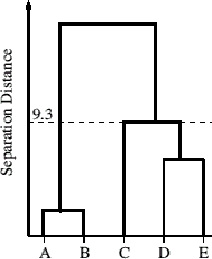Fig. 1: Dendrogram

56. The objects are arranged along the horizontal axis in a convenient order. In this example the letters are purely arbitrary and do not correspond to actual manuscripts. The vertical axis shows the distance between clusters that are linked at a particular level. Here, the distance between the sub-cluster {D, E} and the sub-cluster {C} is 9.3 units.

57. The dendrogram is useful for identifying types, families, and clusters of manuscripts. There is always a single top-level grouping which contains all manuscripts. This all-encompassing group divides into smaller groups that may themselves subdivide at lower levels in the diagram.

58. Agglomerative techniques are the simplest and most often used form of hierarchical cluster analysis. All such techniques employ the same basic process. At the beginning, every cluster contains exactly one object. At each iteration, two clusters are merged to form a larger cluster. The two chosen for merging have the least value of an appropriate criterion for expressing separation between clusters. The dendrogram records the levels at which respective clusters merge, and the process repeats until all of the objects are grouped together in a single cluster.

59. A range of criteria may be used to measure cluster separation. Some require each object's spatial coordinates while others can be calculated directly from the dissimilarity matrix. The four most common techniques used for agglomerative hierarchical clustering are:

1. Nearest Neighbour or Single Linkage Clustering: The distance between two clusters is the distance between the two closest members of the respective clusters.
2. Furthest Neighbour or Complete Linkage Clustering: The distance between clusters is that between the two furthest members of the respective clusters.
3. Mean Distance or Group-Average Clustering: The inter-cluster distance is the average of all distances between members of the respective clusters.
4. Centroid: This method requires a full set of coordinates to be present for all of the objects to be classified. It calculates the centroid coordinates of each cluster, then the Euclidean distances between each pair of centroids. The pair with the least distance is merged before proceeding to the next iteration.Fig. 2: Agglomerative Hierarchical Clustering

60. Centroid clustering requires coordinates for every object. The other three methods can be performed with nothing more than a dissimilarity matrix, making them useful candidates for investigating manuscript relationships.

61. These methods do not necessarily produce the same results. A study by Jardine and Sibson7 suggests that nearest neighbour clustering has a strong theoretical foundation whereas the other methods fall short; however, more recent studies have questioned the validity of Jardine and Sibson's analysis. In practice, mean distance is used more often than nearest neighbour clustering.

62. Unfortunately, nearest neighbour clustering suffers from a tendency to merge groups that are clearly separate apart from a few intermediate objects. This effect is called chaining, and results in a much less clear distinction between clusters than one might otherwise expect. In extreme cases, dissimilar clusters are linked together at a low level.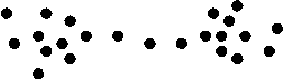Fig. 3: Chaining

63. The furthest neighbour and mean distance methods have been criticised because they tend to favour clusters which are circular (or spherical) in the object space, rather than elliptical clusters with high eccentricities. They also have a tendency to form distinct clusters even when the objects clearly belong to a single cluster. Nevertheless, they are resistant to chaining.

### Conclusion

64. This article surveys multivariate techniques that may be applied to manuscript classification. These techniques are not altogether novel, and have been used in a number of manuscript studies. If used in an uncritical manner, they may produce misleading or even spurious results. Care should be taken to avoid techniques that assume a data model that is not appropriate for manuscripts.

65. Of the techniques examined here, multidimensional scaling and cluster analysis stand out as good candidates for use in manuscript classification studies. They have the potential to make very useful exploratory tools, provided that their limitations are not overlooked.

## Endnotes

1 Gordon D. Fee, "Codex Sinaiticus in the Gospel of John", NTS 15/1 (1968): 23-44.

2 Wieland Willker, "Principal Component Analysis of Manuscripts of the Gospel of John", n.p. [cited 4 March 2002]. Online: http://www-user.uni-bremen.de/~wie/pub/Analysis-PCA.html.

3 Christopher Chatfield and Alexander J. Collins, Introduction to Multivariate Analysis (London: Chapman and Hall, 1980), 190.

4 Chatfield and Collins, Introduction, 201.

5 See, for example, Timothy J. Finney, "The Ancient Witnesses of the Epistle to the Hebrews" (Ph.D. diss., Murdoch University, 1999). Online: http://purl.org/tfinney/thesis.

6 Chatfield and Collins, Introduction, 207.

7 N. Jardine and R. Sibson, Mathematical Taxonomy (New York: Wiley, 1971).

## Bibliography

Chatfield, Christopher, and Alexander J. Collins. Introduction to Multivariate Analysis. London: Chapman and Hall, 1980.

Everitt, B. S. Cluster Analysis. London: Edward Arnold, 1998.

Everitt, B. S., and G. Dunn. Applied Multivariate Data Analysis. London: Edward Arnold, 2000.

Fee, Gordon D. "Codex Sinaiticus in the Gospel of John: A Contribution to Methodology in Establishing Textual Relationships". New Testament Studies 15/1 (1968): 23-44.

Finney, Timothy J. "The Ancient Witnesses of the Epistle to the Hebrews: A Computer-Assisted Analysis of the Papyrus and Uncial Manuscripts of pros ebraious". Ph.D. diss., Murdoch University, 1999. Online http://purl.org/tfinney/thesis.

Griffith, John G. "Numerical Taxonomy and Some Primary Manuscripts of the Gospels". Journal of Theological Studies 20/2 (1969): 389-406.

_______ "Non-stemmatic Classification of Manuscripts by Computer Methods". Colloques internationaux du CNRS 579--La pratique des ordinateurs dans la critique des textes, 74-86.

Jardine, N., and R. Sibson. Mathematical Taxonomy. New York: Wiley, 1971.

Kvalheim, O. M., D. Apollon, and R. H. Pierce. "A Data-analytical Examination of the Claremont Profile Method for Classifying and Evaluating Manuscript Evidence". Symbolae Osloenses 63 (1988): 133-144.

Manly, B. F. J. Multivariate Statistical Methods. London: Chapman and Hall, 1994.

Spencer, Matthew, and Christopher J. Howe. "Estimating Distances between Manuscripts Based on Copying Errors". Literary and Linguistic Computing 16/4 (2001): 467-484.

Willker, Wieland. "Principal Component Analysis of Manuscripts of the Gospel of John". No pages [cited 4 March 2002]. Online: http://www-user.uni-bremen.de/~wie/pub/Analysis-PCA.html.

© 2002 TC: A Journal of Biblical Textual Criticism

http://purl.org/TC/vol07/Thorpe2002.html